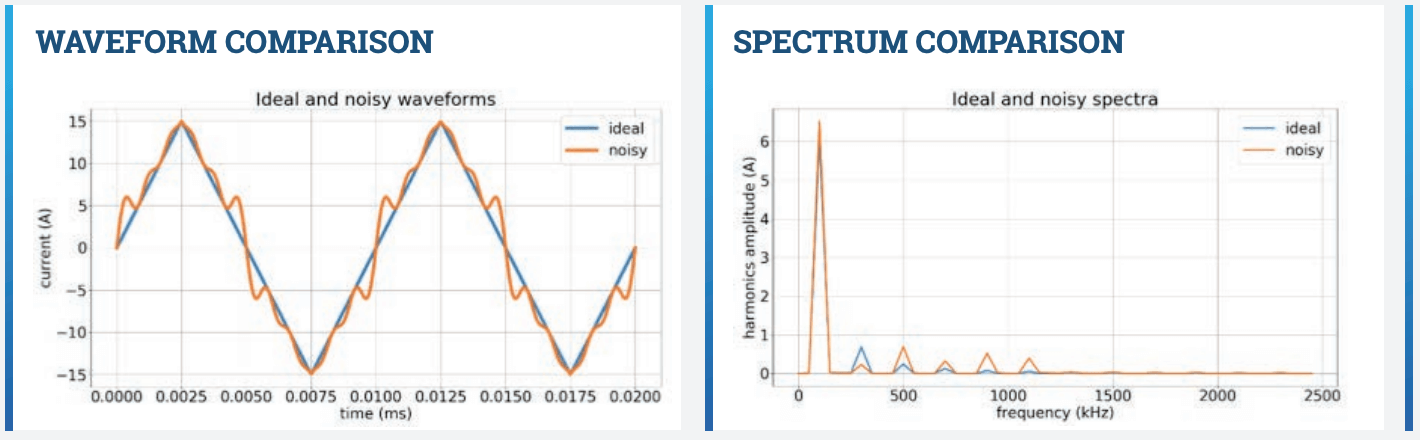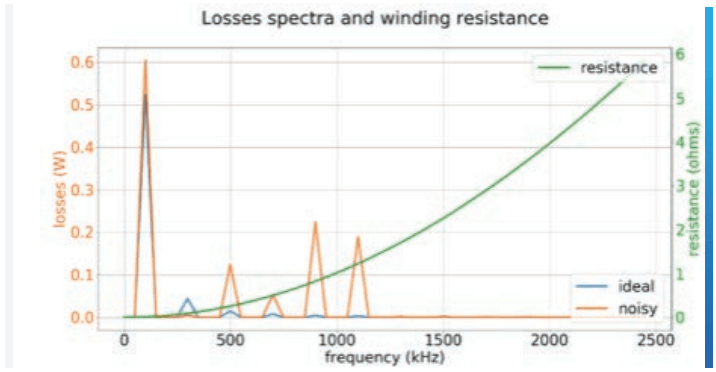# IMPACT ON THE WINDING LOSSES DUE TO THE HARMONICS

Power losses in magnetic components are very frequency-dependent. For this reason, Frenetic technology analyzes the harmonics of the real current waveforms to predict high-frequency losses.

In this document, a comparison of the harmonic analysis is carried out for an ideal triangular waveform and one with noise induced by the converter. The AC resistance is the key factor for the winding losses analysis.

In the waveform comparison, it is appreciated the noisy signal compared with the ideal one and in the spectrum, the harmonics comparison is shown.LOSSES ANALYSIS EXAMPLE

As it is shown in the picture, winding losses are induced by high-frequency harmonics, increasing the losses due to the exponential growth of the AC resistance.

Losses can be drastically reduced when the current waveform approaches its ideal behavior, in this case, the theoretical THD of 12.12% of a triangle wave.CONCLUSIONS

The design of a magnetic component is a very complex task to do it manually, which could produce a number of iterations due to problems nd it during the test stage. Noisy signals are common during rst testing stages, therefore the designs should consider it. Since the technology used by Frenetic is automatized for calculating the losses due to the harmonics, the users of Frenetic can verify the maximum THD that their magnetics could manage before rising maximum temperature.